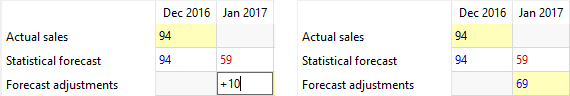# 6.4.1. Formulas in Forecast Adjustments

Forecast adjustments are made via the Table of the Demand forecasting tab. To adjust the forecast, enter desired values into the corresponding cells of the Forecast Adjustments row of the table.

Besides entering concrete values, you can write a brief formulas using the following syntax: `<operation><right value>`. Streamline uses these brief formulas to create final formulas that look like: `<left value><operation><right value>`, where:

• `<left value>` is the Forecast adjustments cell value of the same period.
• `<operation>` is an arithmetic operation that can be one of the following: `+` (plus), `-`(minus), `*`(multiply).
• `<right value>` is the value, entered in the brief formula.

For example, you have Statistical forecast of Jan 2017 as `59`, and want to increase it by `10`. To do this, enter `+10` in the Forecast adjustments cell of this period. Hit Enter. The result of the operation will be displayed in the cell in blue color. Streamline works just like Excel here.To edit a formula, click on the cell (the cell editor will display the formula). The editor allows entering any number of backspaces in any formula position. All of them is removed automatically as you leave the cell.